# PSAT Math : How to find the area of a rectangle

## Example Questions

← Previous 1

### Example Question #1 : Rectangles

A contractor is going to re-tile a rectangular section of the kitchen floor. If the floor is 6ft x 3ft, and he is going to use square tiles with a side of 9in. How many tiles will be needed?

24

2

40

32

32

Explanation:

We have to be careful of our units. The floor is given in feet and the tile in inches. Since the floor is 6ft x 3ft. we can say it is 72in x 36in, because 12 inches equals 1 foot. If the tiles are 9in x 9in we can fit 8 tiles along the length and 4 tiles along the width. To find the total number of tiles we multiply 8 x 4 = 32. Alternately we could find the area of the floor (72 x 36, and divide by the area of the tile 9 x 9)

### Example Question #1 : How To Find The Area Of A Rectangle

The rectangular bathroom floor in Michael’s house is ten feet by twelve feet. He wants to purchase square tiles that are four inches long and four inches wide to cover the bathroom floor. If each square tile costs $2.50, how much money will Michael need to spend in order to purchase enough tiles to cover his entire bathroom floor? Possible Answers:$4800

$1920$5400

$2700$1080

$2700 Explanation: The dimensions for the bathroom are given in feet, but the dimensions of the tiles are given in inches; therefore, we need to convert the dimensions of the bathroom from feet to inches, because we can’t compare measurements easily unless we are using the same type of units. Because there are twelve inches in a foot, we need to multiply the number of feet by twelve to convert from feet to inches. 10 feet = 10 x 12 inches = 120 inches 12 feet = 12 x 12 inches = 144 inches This means that the bathroom floor is 120 inches by 144 inches. The area of Michael’s bathroom is therefore 120 x 144 in2 = 17280 in2. Now, we need to find the area of the tiles in square inches and calculate how many tiles it would take to cover 17280 in2. Each tile is 4 in by 4 in, so the area of each tile is 4 x 4 in2, or 16 in2. If there are 17280 in2 to be covered, and each tile is 16 in2, then the number of tiles we need is 17280 ÷ 16, which is 1080 tiles. The question ultimately asks us for the cost of all these tiles; therefore, we need to multiply 1080 by 2.50, which is the price of each tile. The total cost = 1080 x 2.50 dollars = 2700 dollars. The answer is$2700.

### Example Question #21 : Rectangles

Ron has a fixed length of wire that he uses to make a lot. On Monday, he uses the wire to make a rectangular lot. On Tuesday, he uses the same length of wire to form a square-shaped lot. Ron notices that the square lot has slightly more area, and he determines that the difference between the areas of the two lots is sixteen square units. What is the positive difference, in units, between the length and the width of the lot on Monday?

10

12

4

8

6

8

Explanation:

Let’s say that the rectangular lot on Monday has a length of l and a width of w. The area of a rectangular is the product of the length and the width, so we can write the area of the lot on Monday as lw.

Next, we need to find an expression for the area of the lot on Tuesday. We are told that the lot is in the shape of a square and that it uses the same length of wire. If the length of the wire used is the same on both days, then the perimeter will have to remain the same. In other words, the perimeter of the square will equal the perimeter of the rectangle. The perimeter of a rectangle is given by 2l + 2w.

We also know that if s is the length of a side of a square, then the perimeter is 4s, because each side of the square is congruent. Let’s write an equation that sets the perimeter of the rectangle and the square equal.

2l + 2w = 4s

If we divide both sides by 4 and then simplify the expression, then we can write the length of the square as follows: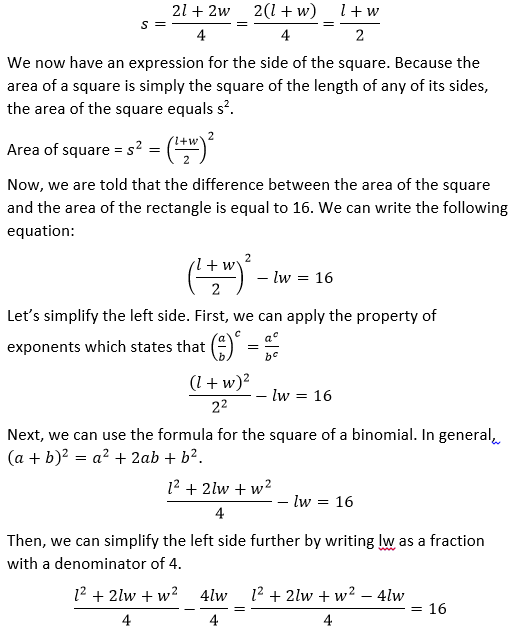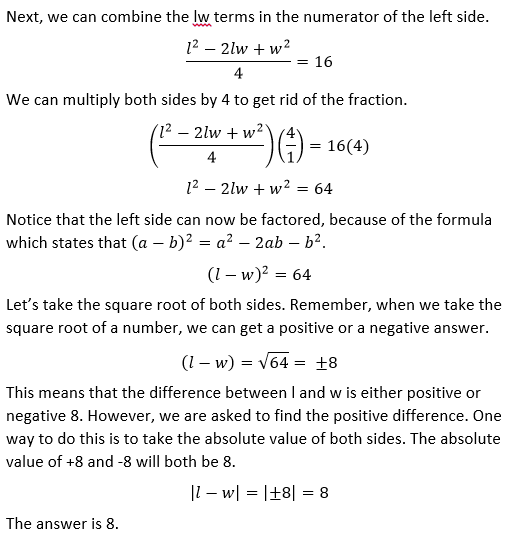### Example Question #11 : Quadrilaterals

A rectangle has a width of 2x.  If the length is five more than 150% of the width, what is the area of the rectangle?

5x + 10

6x2 + 10x

10(x + 1)

6x2 + 5

5x + 5

6x2 + 10x

Explanation:

Given that w = 2x and l = 1.5w + 5, a substitution will show that l = 1.5(2x) + 5 = 3x + 5.

A = lw = (3x + 5)(2x) = 6x2 + 10x

### Example Question #2 : Rectangles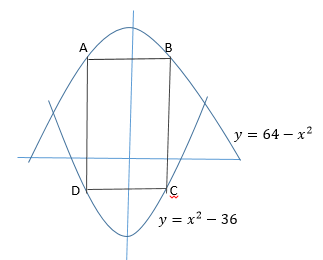Rectangle ABCD is shown in the figure above. Points A and B lie on the graph of y = 64 – x2 , and points C and D lie on the graph of y = x2 – 36. Segments AD and BC are both parallel to the y-axis. The x-coordinates of points A and B are equal to –k and k, respectively. If the value of k changes from 2 to 4, by how much will the area of rectangle ABCD increase?

544

88

272

176

352

176

Explanation: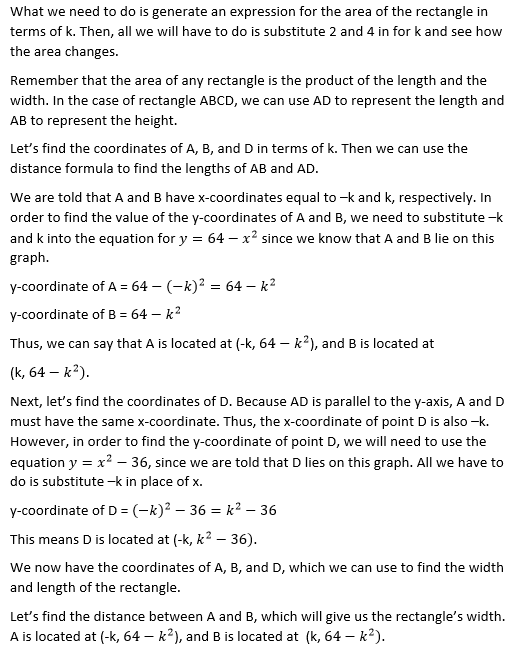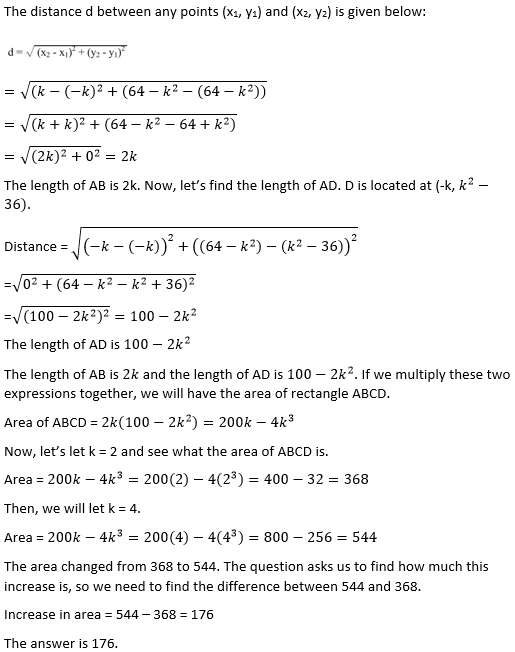### Example Question #3 : New Sat Math No Calculator

George wants to paint the walls in his room blue.  The ceilings are 10 ft tall and a carpet 12 ft by 15 ft covers the floor.  One gallon of paint covers 400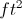and costs $40. One quart of paint covers 100and costs$15.  How much money will he spend on the blue paint?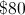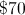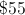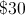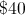Explanation:

The area of the walls is given by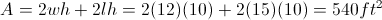One gallon of paint covers 400and the remaining 140would be covered by two quarts.

So one gallon and two quarts of paint would cost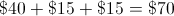### Example Question #181 : Geometry

Daisy gets new carpet for her rectangluar room.  Her floor is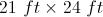.  The carpet sells for \$5 per square yard.  How much did she spend on her carpet?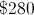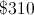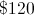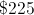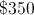Explanation:

Since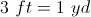the room measurements are 7 yards by 8 yards.  The area of the floor is thus 56 square yards.  It would cost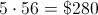.

### Example Question #1 : How To Find The Area Of A Rectangle

The length of a rectangular rug is five more than twice its width.  The perimeter of the rug is 40 ft.  What is the area of the rug?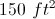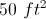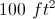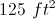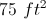Explanation:

For a rectangle,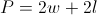and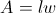where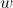is the width andis the length.

Let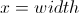and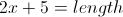.

So the equation to solve becomes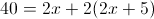or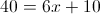.

Thus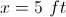and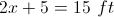, so the area is.

### Example Question #21 : Rectangles

The front façade of a building is 100 feet tall and 40 feet wide.  There are eight floors in the building, and each floor has four glass windows that are 8 feet wide and 6 feet tall along the front façade.  What is the total area of the glass in the façade?

192 ft2

1536 ft2

768 ft2

1536 ft2

2464 ft2

1536 ft2

Explanation:

Glass Area per Window = 8 ft x 6 ft = 48 ft2

Total Number of Windows = Windows per Floor * Number of Floors = 4 * 8 = 32 windows

Total Area of Glass = Area per Window * Total Number of Windows = 48 * 32 = 1536 ft2

### Example Question #321 : Plane Geometry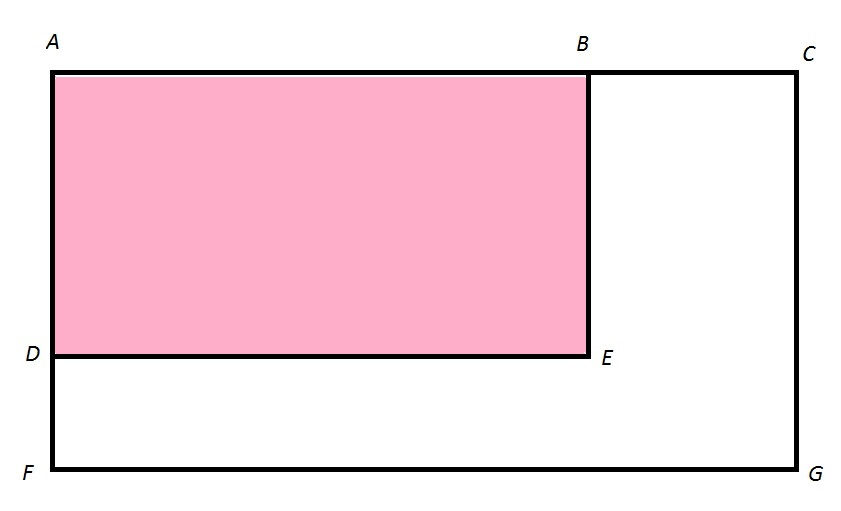Note: Figure NOT drawn to scale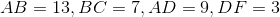What percent of Rectangle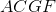is pink?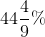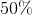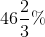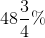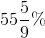Explanation:

The pink region is Rectangle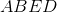. Its length and width are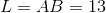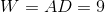so its area is the product of these, or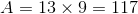.

The length and width of Rectangleare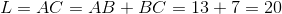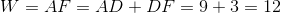so its area is the product of these, or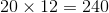.

We want to know what percent 117 is of 240, which can be answered as follows: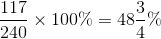← Previous 1

### All PSAT Math Resources